# Periodic Properties

From the discussion of the periodic table, it is evident that those properties which depend upon the electron configuration of an atom will vary periodically with atomic number. On the other hand, those properties which depend upon the total number of electrons will show no such variations. Some of the more common properties which depend upon electronic configurations are:

i) The radius of an atom may be taken as the distance between atomic nucleus and the outermost shell containing electrons of the atom.

ii)  According to the Heisenberg's uncertainty principle the position of a moving electron can not be accurately determined. So the distance between the nucleus and the outermost electron is uncertain.

iii)  Atomic radius can be determined indirectly from the inter nuclear distance between the two atoms in a gaseous diatomic molecule. This internuclear distance between the two atoms is called bond length.

iv)  The inter nuclear distance between the two atoms can be measured by X – ray diffraction or spectroscopic studies.

v)  Covalent radius – One half of the distance between the nuclei (internuclear distance) of two covalently bonded atoms in a homodiatomic molecule is called the covalent radius of that atom. The covalent bond must be single covalent bond. The covalent radius (rA) of atom A in a molecule A2 may be given as: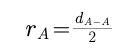i.e. the distance between nuclei of two single covalently bonded atoms in a homodiatomic molecule is equal to the sum of covalent radii of both the atoms.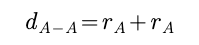In a heterodiatomic molecule AB where the electronegativity of atoms A and B are different, the experimental values of internuclear distance dA-B is less than the theoretical values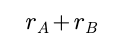According to Schomaker and Stevenson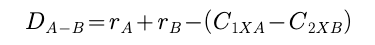C1 and C2 are the Stevenson's coefficients for atoms A and B respectively.

Metal atoms are assumed to be closely packed spheres in the metallic crystal. These metal atom spheres are considered to touch one another in the crystal. One half of the internuclear distance between the two closest metal atoms in the metallic crystal is called metallic radius.

For example – Metallic radius and covalent radius of potassium are 2.3 Å and 2.03Å respectively.

The molecules of non metal atoms are generally gases. On cooling, the gaseous state changes to solid state.

In the solid state, the non metallic elements usually exist as aggregations of molecules are held together by Van der Waal forces. One half of the distance between the nuclei of two adjacent atoms belonging to two neighbouring molecules of a compound in the solid state is called Van der Waal's radius.

It may also be defined as half of the inter nuclear distance of two non bonded neighbouring atoms of two adjacent molecules.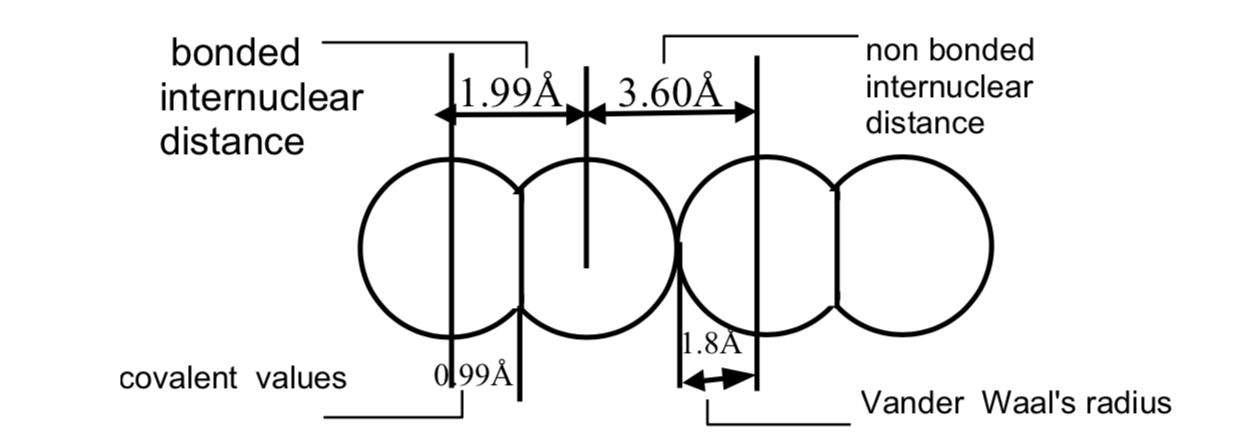The Vander Waal's radius and Covalent radius of Chlorine atom are 1.80Å and 0.99Å respectively

A neutral atom changes to a cation by the loss of one or more electrons and to an anion by the gain of one or more electrons. The number of charge on cation and anion is equal to the number of electrons lost or gained respectively. The ionic radii of the ions present in an ionic crystal may be calculated from the internuclear distance between the two ions

i) Radius of a Cation: Radius of a cation is invariably smaller than that of the corresponding neutral atom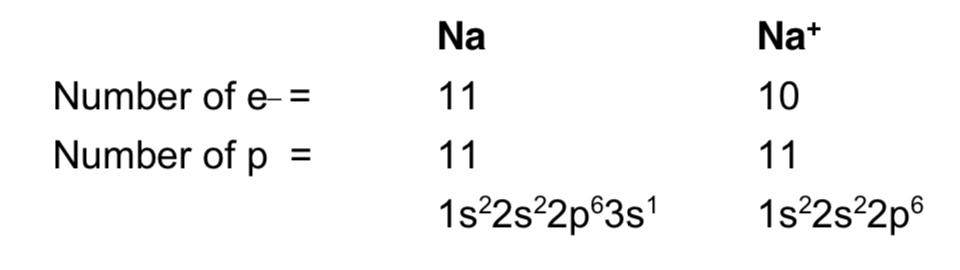Reasons

a) The effective nuclear charge increases. For example in Na atom 11 electrons are attracted by 11 protons and in Na+, 10 electrons are attracted by 11 protons. Thus in the formation of cation number of electrons decreases and nuclear charge remains the same.

b)  Generally the formation of cation results in the removal of the whole outer shell.

c)  Interelectronic repulsion decreases. The interelectronic repulsion in Na is among 11e and in Na+ among 10e

Radius of an anion is invariably bigger than that of the corresponding atom.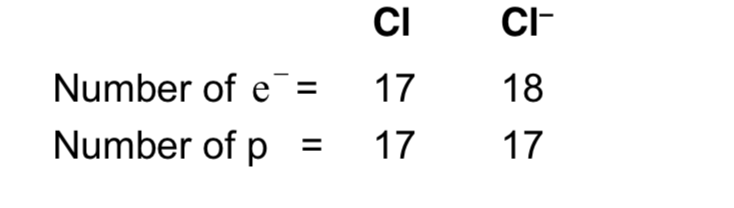Reasons

a)  The effective nuclear charge decrease in the formation of anion. Thus the electrostatic force of attraction between the nucleus and the outer electrons decreases as the size of the anion increases.

b)  Interelectronic repulsion increases.

iii) Isoelectronic series: A series of atoms, ions and molecules in which each species contains same number of electrons but different nuclear charge is called isoelectronic series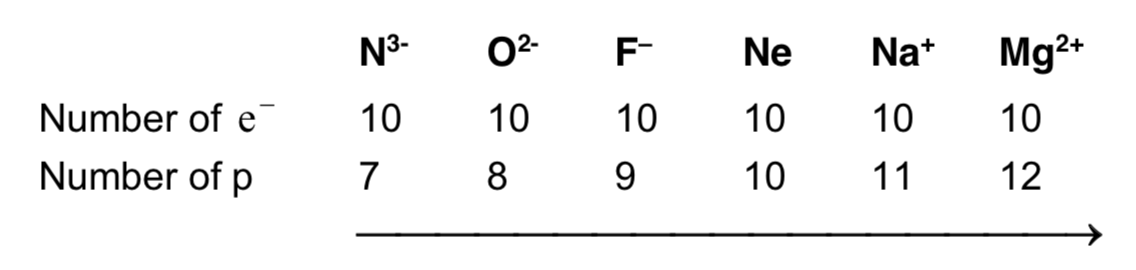a)  Number of electrons is same.

b)  Number of protons is increasing

c)  So the effective nuclear charge is increasing and atomic size is decreasing. In an isoelectronic series atomic size decreases with the increase of charge.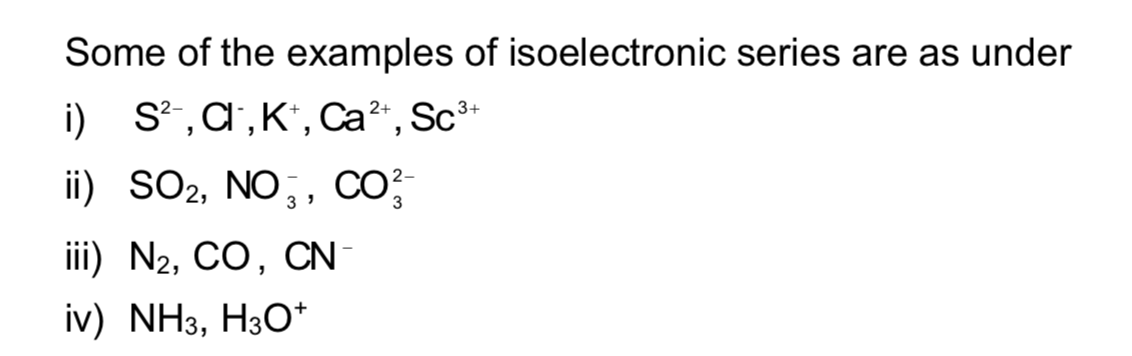Example 1:The radii of Ar is greater than the radii of chlorine

Solution:

In chlorine, the radii means the atomic or covalent radii which are actually half the internuclear distance between 2 atoms whereas in Argon the radii means the Vander Waal’s radii as Argon is not a diatomic molecule.